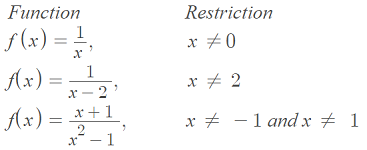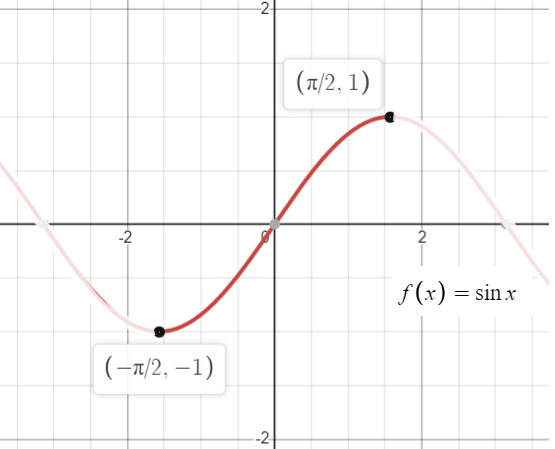# Restrictions of a Function: Definition, Examples

When a function is defined on a smaller set, it’s called a restriction. Reasons to restrict functions include:

1. To formally define the domain of a function.
2. To find an inverse function. For example, trigonometric functions do not have any restrictions, so there is no inverse. However, restricting the domain to a smaller interval allows you to work with inverse functions.
3. To analyze segments that are “better behaved” with bounded variation, better modulus of continuity or monotonicity .

## 1. Restricting Domains to Define Functions

While many functions like the exponential function or cubed root function have no restrictions, others only work on specific sets. Therefore, their formal definitions include one or more restrictions. For example:

Restrictions of a function often happen because of some problem with algebra in the formula. For example, with rational functions, look out for places where the denominator would equal zero:For radical functions, you can’t take square roots of negative numbers:

 Function Restrictions f(x) = √(x + 3). x ≥-3 to avoid the problem of negative numbers. f(x) = &radic(-x) x ≥ 0.

## 2. Restrictions of a Function to Find an Inverse

As an example, if you set an interval to [-π/2, π/2], the sine function becomes one to one :Switching the x and y values of the three coordinates (-π/2, -1), (0, 0), (π/2, 1) gives the inverse: (-1, -π/2), (0, 0), (1, π/2).

## 3. Restriction of a Function to make it “Better Behaved”It’s impossible to pin down a limit for the function sin(1/x) at zero.

Some functions, like f(x) = sin(1/x), behave badly around x = 0. Because the function isn’t defined there, the values oscillate wildly and the function is almost impossible to work with. Restricting the domain to a smaller segment (one that doesn’t include zero!) means that the function is a little more workable.

## References

Graph: Desmos.com
 Restrictions of continuous functions. Retrieved April 2, 2021 from: https://arxiv.org/abs/0711.0679
 Trigonometry With Restrictions. Retrieved May 2, 2021 from: https://www.alamo.edu/contentassets/35e1aad11a064ee2ae161ba2ae3b2559/trigonometric/math2412-inverse-trig-functions.pdf

CITE THIS AS:
Stephanie Glen. "Restrictions of a Function: Definition, Examples" From StatisticsHowTo.com: Elementary Statistics for the rest of us! https://www.statisticshowto.com/restrictions-of-a-function/